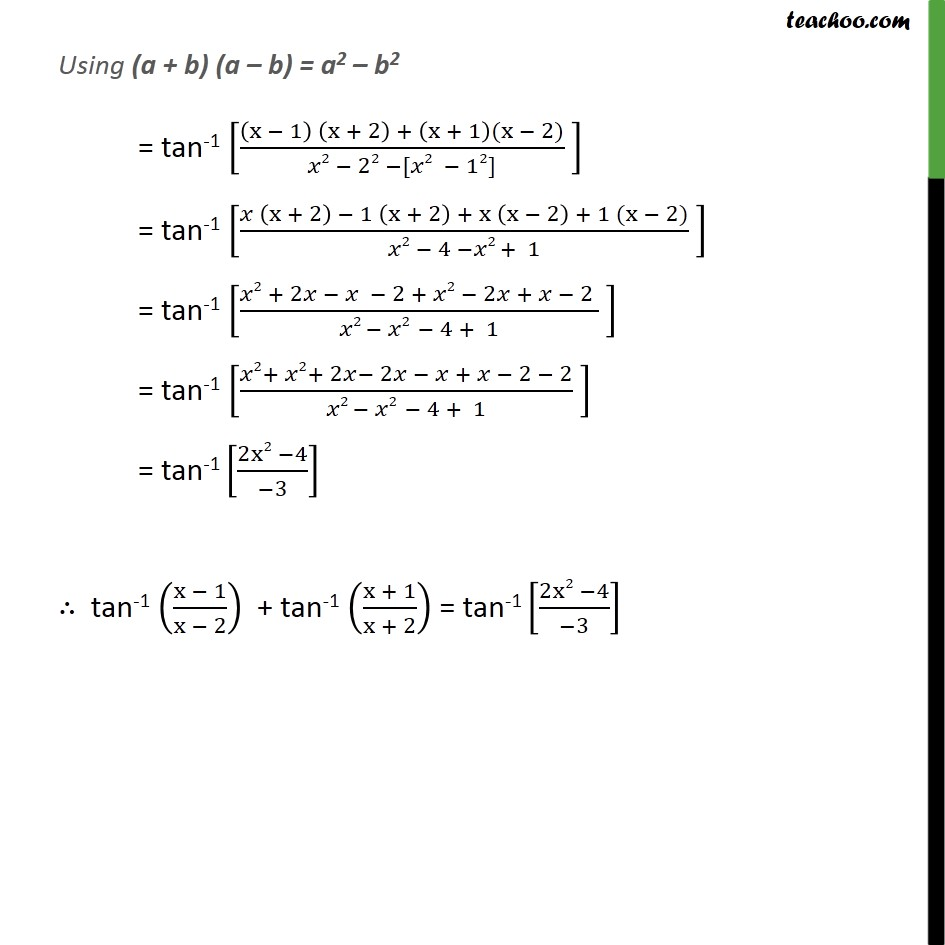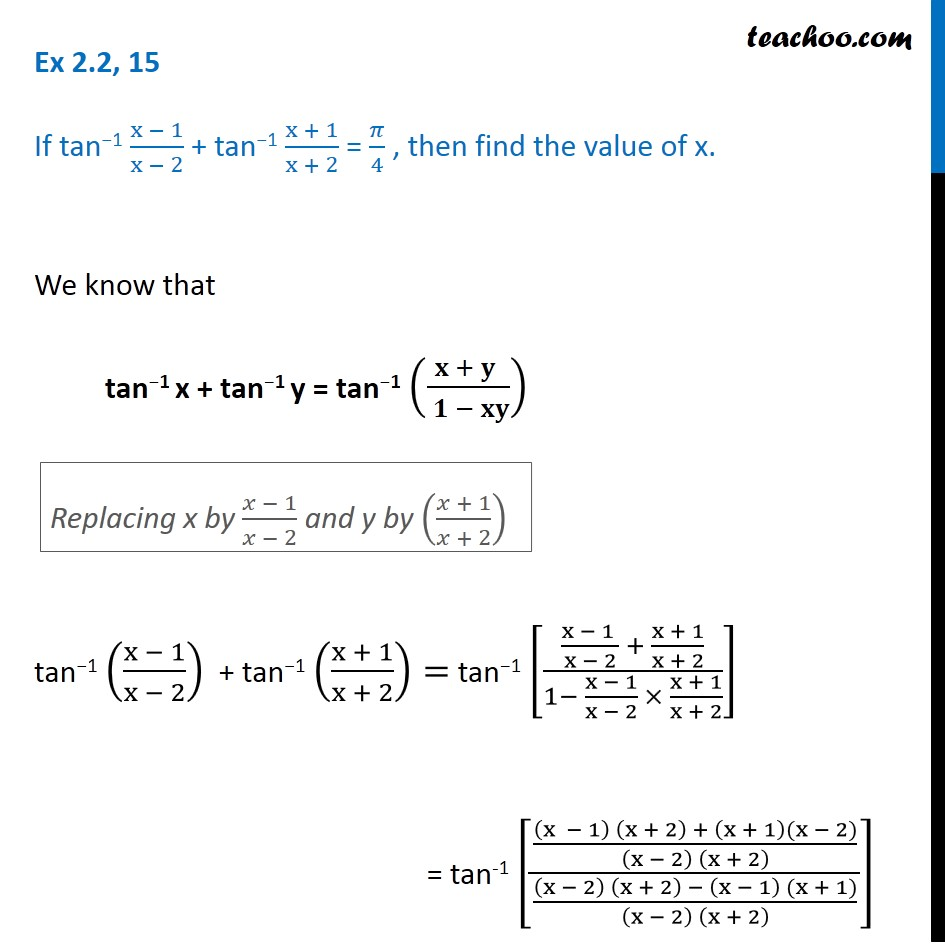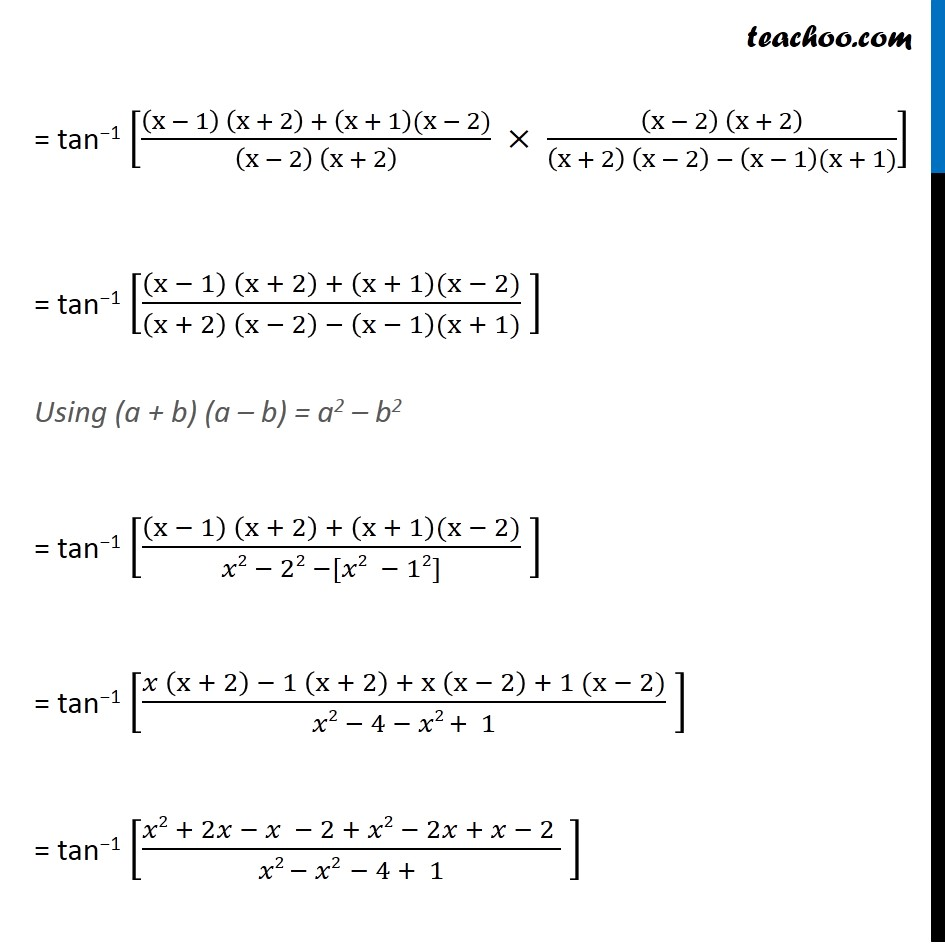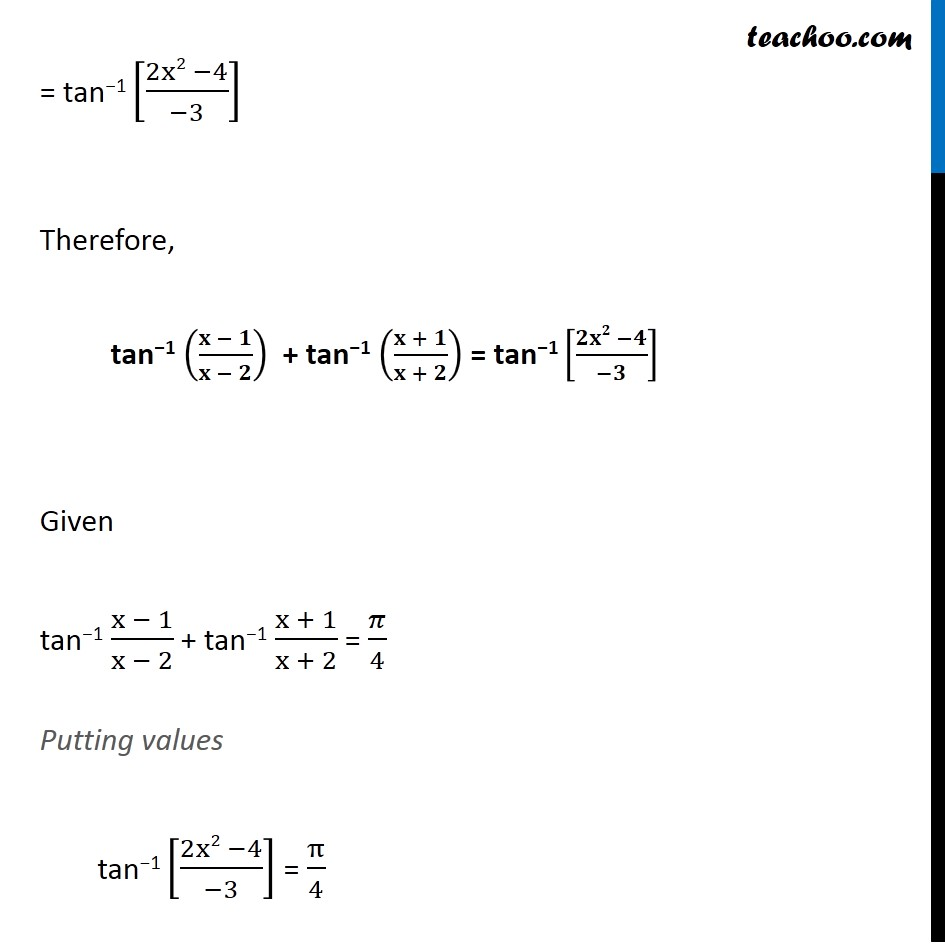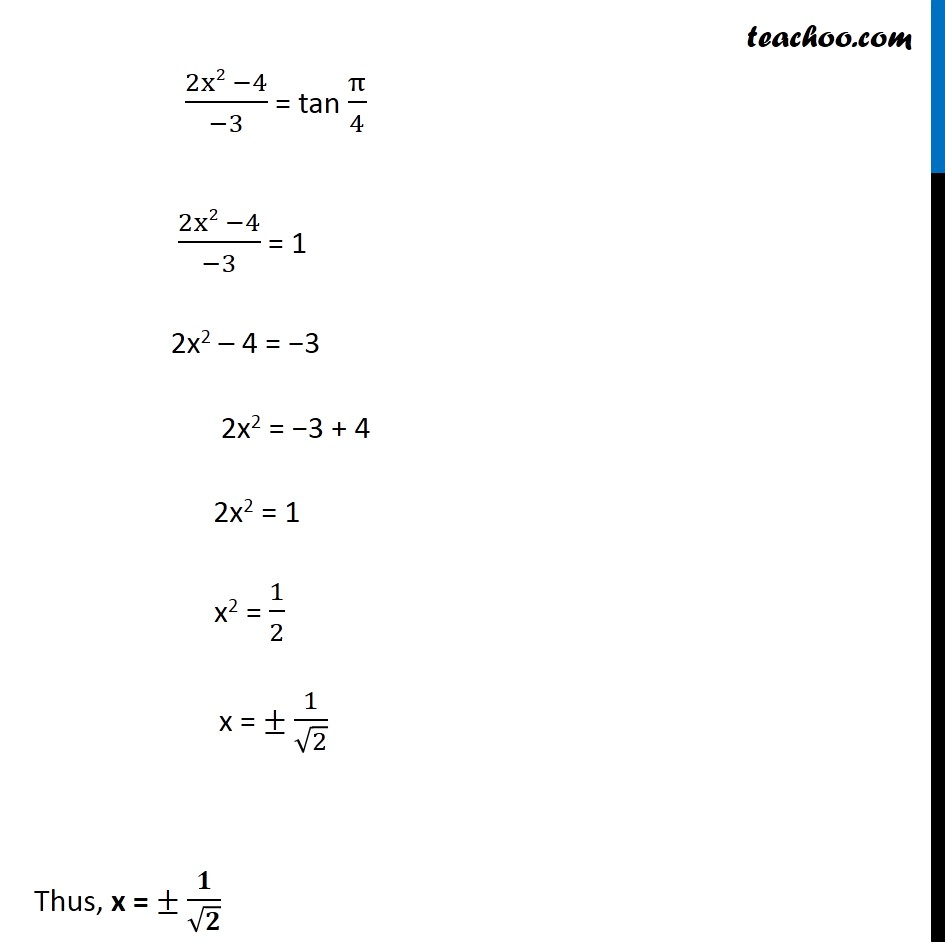Subscribe to our Youtube Channel - https://you.tube/teachoo

1. Chapter 2 Class 12 Inverse Trigonometric Functions
2. Serial order wise
3. Ex 2.2

Transcript

We know that sin"−"1 x + cos"−"1 x = 𝜋/2 sin"−"1 x = 𝜋/2 – cos"−"1 x Replacing x by (𝑥 − 1)/(𝑥 − 2) and y by ((𝑥 + 1)/(𝑥 + 2)) = tan−1 [((x − 1) (x + 2) + (x + 1)(x − 2))/((x − 2) (x + 2) ) × ((x − 2) (x + 2))/((x + 2) (x − 2) − (x − 1)(x + 1))] = tan−1 [((x − 1) (x + 2) + (x + 1)(x − 2))/((x + 2) (x − 2) − (x − 1)(x + 1)) ] Using (a + b) (a – b) = a2 – b2 = tan−1 [((x − 1) (x + 2) + (x + 1)(x − 2))/(𝑥2 − 22 −[𝑥2 − 12]) ] = tan−1 [(𝑥 (x + 2) − 1 (x + 2) + x (x − 2) + 1 (x − 2))/(𝑥2 − 4 − 𝑥2 + 1) ] = tan−1 [(𝑥2 + 2𝑥 − 𝑥 − 2 + 𝑥2 − 2𝑥 + 𝑥 − 2 )/(𝑥2 − 𝑥2 − 4 + 1) ] = tan−1 [(2x2 −4)/(−3)] Therefore, tan−1 ((𝐱 − 𝟏)/(𝐱 − 𝟐)) + tan−1 ((𝐱 + 𝟏)/(𝐱 + 𝟐)) = tan−1 [(𝟐𝐱𝟐 −𝟒)/(−𝟑)] Given tan−1 (x − 1)/(x − 2) + tan−1 (x + 1)/(x + 2) = 𝜋/4 Putting values tan−1 [(2x2 −4)/(−3)] = π/4 (2x2 −4)/(−3) = tan π/4 (2x2 −4)/(−3) = 1 2x2 – 4 = −3 2x2 = −3 + 4 2x2 = 1 x2 = 1/2 x = ± 1/√2 Thus, x = ± 𝟏/√𝟐

Ex 2.2